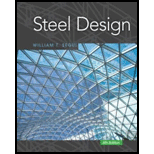# The tension member shown in Figure P3.2-5 must resist a service dead load of 25 kips and a service live load of 45 kips. Does the member have enough strength? The steel is A588, and the bolts are 1 1 8 inches in diameter. Assume that A e = A n . a . Use LRFD. b . Use ASD.### Steel Design (Activate Learning wi...

6th Edition
Segui + 1 other
Publisher: Cengage Learning
ISBN: 9781337094740

#### Solutions

Chapter
Section### Steel Design (Activate Learning wi...

6th Edition
Segui + 1 other
Publisher: Cengage Learning
ISBN: 9781337094740
Chapter 3, Problem 3.2.5P
Textbook Problem
5 views

## The tension member shown in Figure P3.2-5 must resist a service dead load of 25 kips and a service live load of 45 kips. Does the member have enough strength? The steel is A588, and the bolts are 1 1 8 inches in diameter. Assume that A e = A n .a. Use LRFD.b. Use ASD.To determine

(a)

If the member has enough strength using Load and Resistance Factor Design (LRFD).

### Explanation of Solution

Given:

A588 steel of 38×712 tension member with three 118 diameter bolt.

The effective area Ae=An.

Concept Used:

Write the expression for the factored strength in yielding.

P=Φy×Pny …… (I)

Write the expression for the factored strength in rupture.

P=Φf×Pnf …… (II)

Here the strength of the material is P, the resistance factor for yielding is Φy, the resistance factor for rupture is Φf, the nominal strength in yielding is Pny and the nominal strength in rupture is Pnr

The design strength of LRFD is the minimum of Equation (I) and Equation (II).

Write the expression for the nominal strength in yielding.

Pny=Fy×Ag …… (III)

Here, the yield strength in yielding is Fy, the gross area of the member is Ag.

Write the expression for the gross area of the member.

Ag=L×t …… (IV)

Here, the length of the member is L, the thickness of the member is t.

Write the expression for the nominal strength in rupture.

Pnf=Fu×Ae …… (V)

Here, the yield strength in rupture is Fu, the effective area is Ae.

Given that, Ae=An,

Ae=An=AgAholes …… (VI)

Here, the area of the holes is Aholes, the net area is An

Write the expression for the area of the holes.

Aholes=t×dholes …… (VII)

Here, the diameter of the holes is dholes.

Write the expression for the diameter of the holes.

dholes=d+316 …… (VIII)

Here, the diameter of the bolts is d and 316 is the allowance for side clearance for bolts greater than 1.

For the A36 steel resistance factors and the yield strength values are,

Φy=0.90Φf=0.75Fy=50ksiFu=70ksi

Calculation:

Calculate the diameter of holes.

Substitute 118in for d in Equation (VIII).

dholes=118in+316in=1.313in

Calculate the gross area.

Substitute 712in for L and 38in for t in Equation (IV)

To determine

(b)

If the member has enough strength.

### Still sussing out bartleby?

Check out a sample textbook solution.

See a sample solution

#### The Solution to Your Study Problems

Bartleby provides explanations to thousands of textbook problems written by our experts, many with advanced degrees!

Get Started

Find more solutions based on key concepts
As explained in earlier chapters, fins, or extended surfaces, commonly are used in a variety of engineering app...

Engineering Fundamentals: An Introduction to Engineering (MindTap Course List)

The force system acting on the plate is equivalent to a single couple C. Determine.

International Edition---engineering Mechanics: Statics, 4th Edition

What is the purpose of an ISSP?

Management Of Information Security

Which two tool posts are the most efficient if several tools are to be used?

Precision Machining Technology (MindTap Course List)

Do you have multiple user accounts on your computer? Why or why not?

Enhanced Discovering Computers 2017 (Shelly Cashman Series) (MindTap Course List)

If your motherboard supports ECC DDR3 memory, can you substitute non-ECC DDR3 memory?

A+ Guide to Hardware (Standalone Book) (MindTap Course List)

Can all electrodes be used with a leading angle? Why or why not?

Welding: Principles and Applications (MindTap Course List)